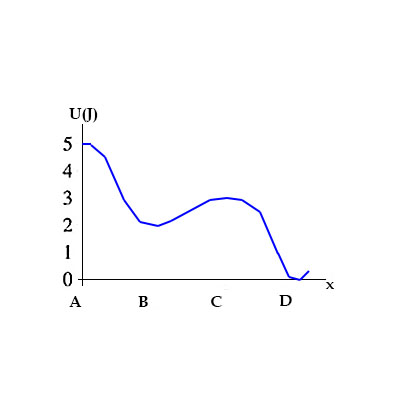# In the figure provided, a 25 g particle is released from rest at x=A. What is the speed of the...

## Question:

In the figure provided, a {eq}25 g {/eq} particle is released from rest at {eq}x=A {/eq}. What is the speed of the particle at {eq}x =D? {/eq}## Conservation of Energy:

The total energy of an isolated object remains constant at all times until an external force acts on it. For example, a falling object loses its potential energy as it gets closer to the ground, but at the same time, it gains kinetic energy due to its acceleration towards the ground resulting in the increase of it speed. The change in the potential energy has the same magnitude as the change in the kinetic energy, keeping the total energy of the falling object constant.

In our case, we have a particle that is released from a point A where its potential energy is

{eq}U_i \ = \ 5 \ J {/eq}

Note that the particle is initially at rest, hence it initial kinetic energy is

{eq}K.E \ = \ 0 \ J {/eq}

At the point D, the potential energy of the particle is now

{eq}U_f \ = \ 0 \ J {/eq}

Form conservation of energy, the kinetic energy of the particle at point D is

{eq}K.E_f \ = \ |U_f \ - \ U_i| \ = \ 5 \ J {/eq}

From the definition of kinetic energy, this is given by the exression

{eq}K.E_f \ = \ 0.5 \ \times \ \dfrac{25 \ g}{1000 \ g/kg} \ \times v^2 \ = \ 0.0125 \ kg \ \times \ v^2 \ = \ 5 \ J {/eq}

where v is the speed of the particle at point D. Solving for the speed v, we get

{eq}v \ = \ \sqrt{\dfrac{5 \ J}{0.0125 \ kg}} \ = \ \mathbf{20 \ m/s} {/eq}